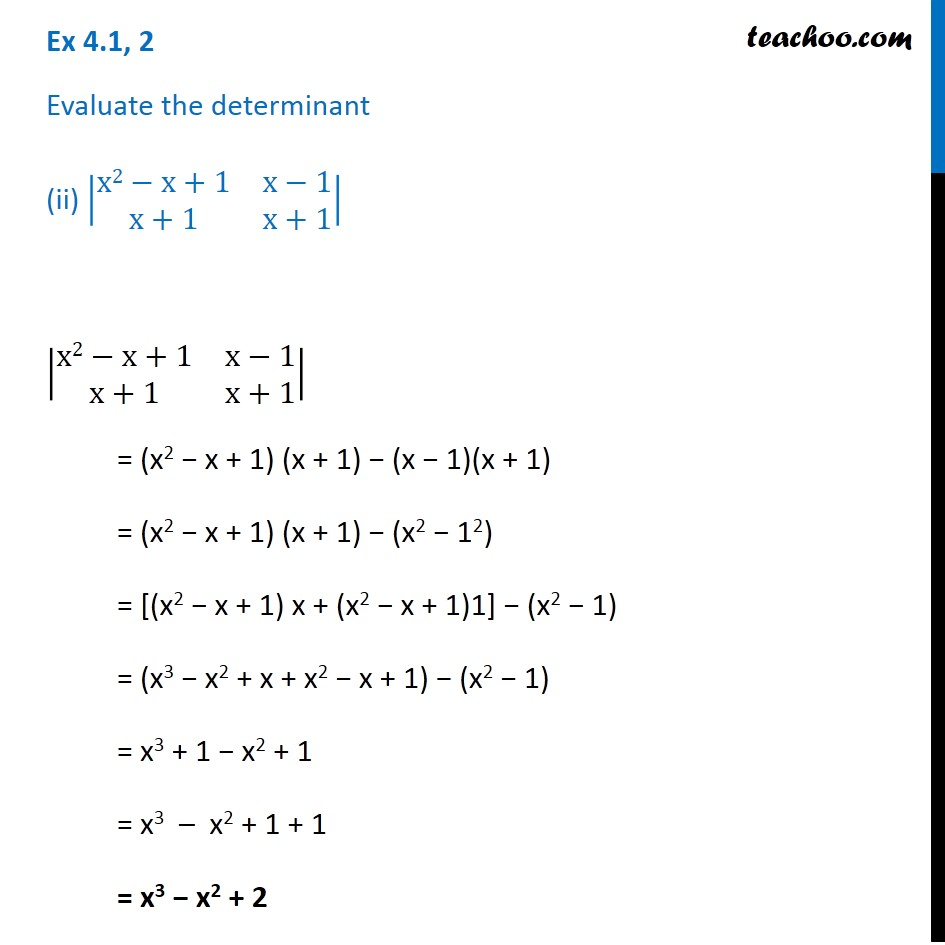Ex 4.1

Chapter 4 Class 12 Determinants
Serial order wiseLearn in your speed, with individual attention - Teachoo Maths 1-on-1 Class

### Transcript

Ex 4.1, 2 Evaluate the determinant (ii) |■8(x2−x+1&x−[email protected]+1&x+1)| |■8(x2−x+1&x−[email protected]+1&x+1)| = (x2 − x + 1) (x + 1) − (x − 1)(x + 1) = (x2 − x + 1) (x + 1) − (x2 − 12) = [(x2 − x + 1) x + (x2 − x + 1)1] − (x2 − 1) = (x3 − x2 + x + x2 − x + 1) − (x2 − 1) = x3 + 1 − x2 + 1 = x3 – x2 + 1 + 1 = x3 − x2 + 2